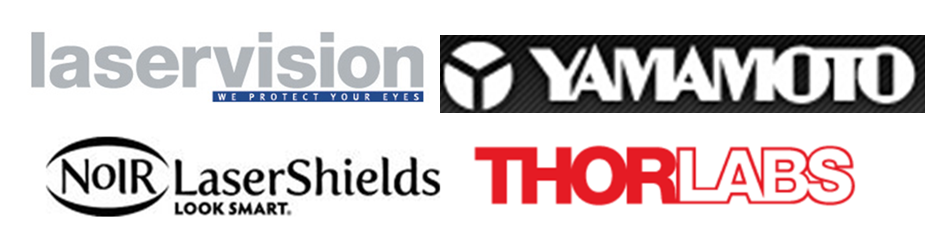English购物车网站地图

## 激光护目镜的主流品牌Laservision是激光护目镜的一线品牌，拥有强悍的技术实力，多个型号的激光防护眼镜的防护等级达到OD10+/9+，性能参数相对其它公司有不小的优势，同时价格也相对高些，主要针对高端用户的需求。

Thorlabs激光护目镜被很多科研客户广泛采用，在高校和研究所中享有很高的知名度，其产品型号和镜架都是经过优选的，虽然型号只有十几款，但基本能覆盖用户的主流需求。在品牌方面Thorlabs是以收购为主，激光防护眼镜只是一个边缘产品。

海纳光学代理LaservisionNOIR公司的激光护目镜，同时为了方便科研用户的选型，我们还进行了美国Thorlabs激光护目镜和美国NOIR公司激光防护眼镜的型号替代分析，下面是替换表格。

 Thorlabs的型号 Noir的型号 防护等级，OD值 可见光透过率VLT LG1 LG1A LG1B YG3 190 to 400 nm, OD = 5+ 808 to 840 nm, OD = 4+ >840 to 950 nm, OD = 5+ >950 to 1080 nm, OD = 7+ >1080 to 1090 nm, OD = 5+ 59% LG2 LG2A LG2B IRD 190 to 450 nm, OD = 6+ 770 to 1800 nm, OD = 2+ 820 to 1720 nm, OD = 3+ 870 to 1600 nm, OD = 4+ 940 to 1070 nm, OD = 5+ 19% LG3 LG3A LG3B ARG 180 to 532 nm, OD = 7+ 48% LG4 LG4A LG4B DI4 190 to 400 nm, OD = 5+ 625 to 850 nm, OD = 4+ 662 to 835 nm, OD = 5+ 633 nm, OD = 5+ LG4: 12% DI4: 14% LG5 LG5A LG5B DI2 180 to 390 nm, OD = 6+ 785 to 830 nm, OD = 5+ 800 to 818 nm, OD = 6+ 61% LG6 LG6A LG6B EC2 190 to 398 nm, OD = 7+ 9,000 to 11,000 nm, OD = 7+ 93% LG7 LG7A LG7B RB2 190 to 400 nm, OD = 6+ 615 to 720 nm, OD = 3+ 651 to 670 nm, OD = 4+ 671 to 715 nm, OD = 5+ 680 to 710 nm, OD = 6+ 690 to 700 nm, OD = 7+ 35% LG8 LG8A LG8B DI6 610 to 664 nm, OD = 1+ 665 to <670 nm, OD = 2+ 670 to 690 nm, OD = 3+ >690 to 698 nm, OD = 2+ 735 to 789 nm, OD = 1+ 790 to <808 nm, OD = 2+ 808 to 1050 nm, OD = 3+ >1050 to 1080 nm, OD = 2+ 35% LG9 LG9A LG9B YG2 180 to 400 nm, OD = 6+ 720 to 1090 nm, OD = 5+ 750 to 1064 nm, OD = 7+ 25% LG10 LG10A LG10B DBY 190 to 534 nm, OD = 7+ 850 to 925 nm, OD = 5+ 925 to 1070 nm, OD = 6+ 960 to 1064 nm, OD = 7+ 35% LG11 LG11A FG1 850 to 2800 nm, OD = 3+ 900 to 2600 nm, OD = 4+ 950 to 1010 nm, OD = 5+ >1010 to 1500 nm, OD = 7+ >1500 to 2350 nm, OD = 5+ >2800 to 10,600 nm, OD = 5+ 75% LG12 LG12A LG12B YAD 180 to 534 nm, OD = 7+ 720 to 730 nm, OD = 5+ >730 to 740 nm, OD = 6+ >740 to 1070 nm, OD = 7+ 11% LG13 LG13A LG13B DIA 190 to 380 nm, OD = 5+ 630 to 700 nm, OD = 1.5+ 39% LG14 LG14A LG14B AG3 190 to 400 nm, OD = 5+ 518 to 523 nm, OD = 2+ 524 to 532 nm, OD = 3+ 533 to 536 nm, OD 2+ 47% LG15 LG15A LG15B DYE 190 to 400 nm, OD = 5+ 576 to 600 nm, OD = 5+ 582 to 598 nm, OD = 6+ 585 to 595 nm, OD = 7+ 15% LG16 LG16A LG16B HOY 190 to 400 nm, OD = 5+ 1450 to 1650 nm, OD = 2+ >1650 to 1775 nm, OD = 3+ >1775 to 3000 nm, OD = 4+ 1980 to 2550 nm, OD = 5+ 5200 to 11000 nm, OD = 5+ 9000 to 10600 nm, OD = 6+ LG16: 41% HOY: 53%